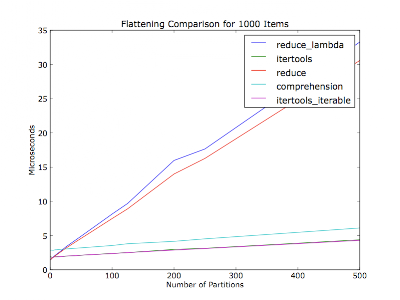Flattening a shallow list in Python [duplicate] Flattening a shallow list in Python [duplicate] python python

# Flattening a shallow list in Python [duplicate]

If you're just looking to iterate over a flattened version of the data structure and don't need an indexable sequence, consider itertools.chain and company.

``>>> list_of_menuitems = [['image00', 'image01'], ['image10'], []]>>> import itertools>>> chain = itertools.chain(*list_of_menuitems)>>> print(list(chain))['image00', 'image01', 'image10']``

It will work on anything that's iterable, which should include Django's iterable `QuerySet`s, which it appears that you're using in the question.

Edit: This is probably as good as a reduce anyway, because reduce will have the same overhead copying the items into the list that's being extended. `chain` will only incur this (same) overhead if you run `list(chain)` at the end.

Meta-Edit: Actually, it's less overhead than the question's proposed solution, because you throw away the temporary lists you create when you extend the original with the temporary.

Edit: As J.F. Sebastian says `itertools.chain.from_iterable` avoids the unpacking and you should use that to avoid `*` magic, but the timeit app shows negligible performance difference.

You almost have it! The way to do nested list comprehensions is to put the `for` statements in the same order as they would go in regular nested `for` statements.

Thus, this

``for inner_list in outer_list:    for item in inner_list:        ...``

corresponds to

``[... for inner_list in outer_list for item in inner_list]``

So you want

``[image for menuitem in list_of_menuitems for image in menuitem]``

@S.Lott: You inspired me to write a timeit app.

I figured it would also vary based on the number of partitions (number of iterators within the container list) -- your comment didn't mention how many partitions there were of the thirty items. This plot is flattening a thousand items in every run, with varying number of partitions. The items are evenly distributed among the partitions.Code (Python 2.6):

``#!/usr/bin/env python2.6"""Usage: %prog item_count"""from __future__ import print_functionimport collectionsimport itertoolsimport operatorfrom timeit import Timerimport sysimport matplotlib.pyplot as pyplotdef itertools_flatten(iter_lst):    return list(itertools.chain(*iter_lst))def itertools_iterable_flatten(iter_iter):    return list(itertools.chain.from_iterable(iter_iter))def reduce_flatten(iter_lst):    return reduce(operator.add, map(list, iter_lst))def reduce_lambda_flatten(iter_lst):    return reduce(operator.add, map(lambda x: list(x), [i for i in iter_lst]))def comprehension_flatten(iter_lst):    return list(item for iter_ in iter_lst for item in iter_)METHODS = ['itertools', 'itertools_iterable', 'reduce', 'reduce_lambda',           'comprehension']def _time_test_assert(iter_lst):    """Make sure all methods produce an equivalent value.    :raise AssertionError: On any non-equivalent value."""    callables = (globals()[method + '_flatten'] for method in METHODS)    results = [callable(iter_lst) for callable in callables]    if not all(result == results for result in results[1:]):        raise AssertionErrordef time_test(partition_count, item_count_per_partition, test_count=10000):    """Run flatten methods on a list of :param:`partition_count` iterables.    Normalize results over :param:`test_count` runs.    :return: Mapping from method to (normalized) microseconds per pass.    """    iter_lst = [[dict()] * item_count_per_partition] * partition_count    print('Partition count:    ', partition_count)    print('Items per partition:', item_count_per_partition)    _time_test_assert(iter_lst)    test_str = 'flatten(%r)' % iter_lst    result_by_method = {}    for method in METHODS:        setup_str = 'from test import %s_flatten as flatten' % method        t = Timer(test_str, setup_str)        per_pass = test_count * t.timeit(number=test_count) / test_count        print('%20s: %.2f usec/pass' % (method, per_pass))        result_by_method[method] = per_pass    return result_by_methodif __name__ == '__main__':    if len(sys.argv) != 2:        raise ValueError('Need a number of items to flatten')    item_count = int(sys.argv)    partition_counts = []    pass_times_by_method = collections.defaultdict(list)    for partition_count in xrange(1, item_count):        if item_count % partition_count != 0:            continue        items_per_partition = item_count / partition_count        result_by_method = time_test(partition_count, items_per_partition)        partition_counts.append(partition_count)        for method, result in result_by_method.iteritems():            pass_times_by_method[method].append(result)    for method, pass_times in pass_times_by_method.iteritems():        pyplot.plot(partition_counts, pass_times, label=method)    pyplot.legend()    pyplot.title('Flattening Comparison for %d Items' % item_count)    pyplot.xlabel('Number of Partitions')    pyplot.ylabel('Microseconds')    pyplot.show()``

Edit: Decided to make it community wiki.

Note: `METHODS` should probably be accumulated with a decorator, but I figure it'd be easier for people to read this way.